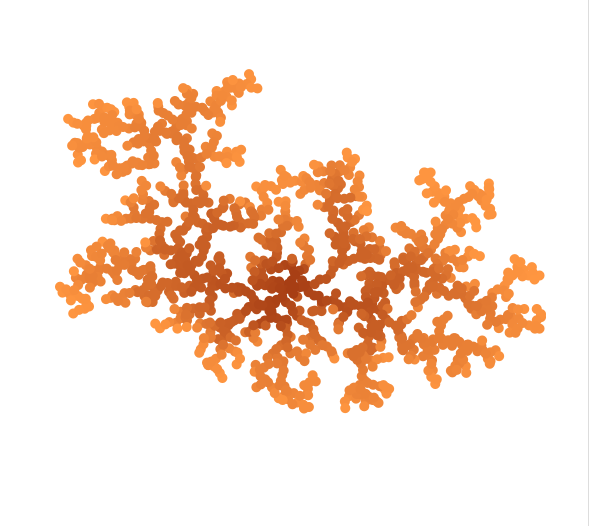## corral structures from particle collisions

I wrote a processing sketch that creates 2D corral like structures, by throwing a group of particles at a center location and making them stick whenever they hit another already sticked particlethe sketch starts by initializing a sticky particle at the seed location and then placing all other particles at random locations with their direction vector pointing to the seed particle

then each particle moves in the direction of the center and if it hits an already sticky particle it gets sticky as well. When two nonsticky particles collide, they get shifted around a to a random position to prevent them creating endless loops.

Here is the main source code

``````Particle[] particles = new Particle;
PVector seed;
void setup() {
colorMode(RGB);
size(600, 600);
seed = new PVector(300,300);
for (int i=0; i<particles.length; i++) {
PVector pos = new PVector(random(width), random(height));
PVector dir = PVector.sub(pos, seed);
dir = dir.mult(-0.01);
particles[i] = new Particle(pos, dir);
}
particles = new Particle(seed, new PVector(0, 0));
frameRate(25);
}

void draw() {
background(255);
for (int i=0; i<particles.length; i++) {
particles[i].draw();

if (particles[i].die(particles)) {
}
if (particles[i].collide(particles)) {
particles[i].collision = true;
PVector pos = PVector.add(particles[i].pos, new PVector(random(-5, 5), random(-5, 5)));
PVector dir = PVector.sub(pos, seed);
dir = dir.mult(-0.01);
particles[i].dir = dir;
particles[i].pos = pos;
}
}
particles[i].update();
}
}
``````

And here is the source code of the particle class

``````class Particle {
PVector pos;
PVector dir;
boolean collision = false;
int age;
Particle(PVector pos, PVector dir) {
this.pos = pos;
this.dir = dir;
this.age =0;
}

void draw() {
fill(255-age/2, 150-age/2, 64-age/4);
noStroke();
} else {
fill(200,100);
stroke(0,100);
}
if (dead)  ellipse(pos.x, pos.y, 10, 10);

}

void update() {
this.dir = PVector.sub(pos, new PVector(300, 300));
this.dir = dir.mult(-0.01);
}
age++;
if ( age > 255) {
age = 255;
}
}
collision = false;
}

boolean die( Particle[] others ) {
for ( int i=0; i<others.length; i++) {
if (others[i] != this) {
if (PVector.dist(others[i].pos, this.pos) < 7 && others[i].dead) {
return true;
}
}
}
return false;
}

boolean collide( Particle[] others ) {
for ( int i=0; i<others.length; i++) {
if (others[i] != this) {
if (PVector.dist(others[i].pos, this.pos) < 11) {
return true;
}
}
}
return false;
}

}
``````